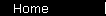### Beta

A measure of an investment's volatility, relative to an appropriate asset class. For stocks, the asset class is usually taken to be the S&P 500 index.

The formula is:

beta   =   [ Cov(r, Km) ] / [ StdDev(Km) ]2
where
r is the return rate of the investment;
Km is the return rate of the asset class.

If the asset class is well chosen so that the return fluctuations of the investment and the class are highly correlated, then the formula approximates "the volatility of the investment divided by the volatility of the class."

Beta is used in modern portfolio theory as a measure of risk; it's specifically used in the Capital Asset Pricing Model.

See the main pages on CAPM and CAPM regression.

 home  |  glossary  |  calculator  |  about us  |  books